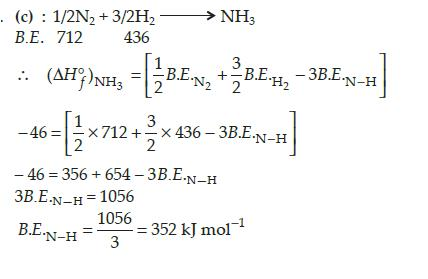Courses

# Test: Previous Year Questions: Thermochemistry

## 12 Questions MCQ Test Chemistry Class 11 | Test: Previous Year Questions: Thermochemistry

Description
This mock test of Test: Previous Year Questions: Thermochemistry for JEE helps you for every JEE entrance exam. This contains 12 Multiple Choice Questions for JEE Test: Previous Year Questions: Thermochemistry (mcq) to study with solutions a complete question bank. The solved questions answers in this Test: Previous Year Questions: Thermochemistry quiz give you a good mix of easy questions and tough questions. JEE students definitely take this Test: Previous Year Questions: Thermochemistry exercise for a better result in the exam. You can find other Test: Previous Year Questions: Thermochemistry extra questions, long questions & short questions for JEE on EduRev as well by searching above.
QUESTION: 1

### If at 298 K the bond energies of C–H, C–C, C=C and H–H bonds are respectively 414, 347, 615 and 435 kJ mol-1, the value of enthalpy change for the reaction ; H2C = CH2(g) + H2(g) → H3C–CH3(g) at 298 K will be –                [AIEEE-2003]

Solution:

ΔHReaction = ∑BE Reactant - ∑BE Product

= 4 x 414 + 615 + 435 – (6 x 414 + 347)

= 2706 – 2831

= –125 kJ

QUESTION: 2

### The enthalpy change for a reaction does not depend upon - [AIEEE-2003]

Solution:

Hess's Law of Constant Heat Summation states that regardless of the multiple stages or intermediate steps of a reaction, the total enthalpy change for the reaction is the sum of all changes.

The enthalpy of a chemical process is independent of the path taken from the initial to the final state.

QUESTION: 3

### The enthalpies of combustion of carbon and carbon monoxide are -393.5 and -283 kJ mol-1 respectively. The enthalpy of formation of carbon monoxide per mole -   [AIEEE-2004]

Solution:

C + O2  → CO2
∆Hc = -393.5 kJ/mol    -i
CO + ½ O2  → CO2
∆Hc = -283 kJ/mol      -ii
On i-ii
We get
C + 1/2 O2  → CO
∆Hf = -110.5 kJ/mol

QUESTION: 4

If the bond dissociation energies of XY, X2 and Y2 (all diatomic molecules) are in the ratio of  1 : 1 : 0.5 and ΔfH for the formation of XY is -200 kJ mol-1. The bond dissociation energy of X2 will be

[AIEEE-2005]

Solution: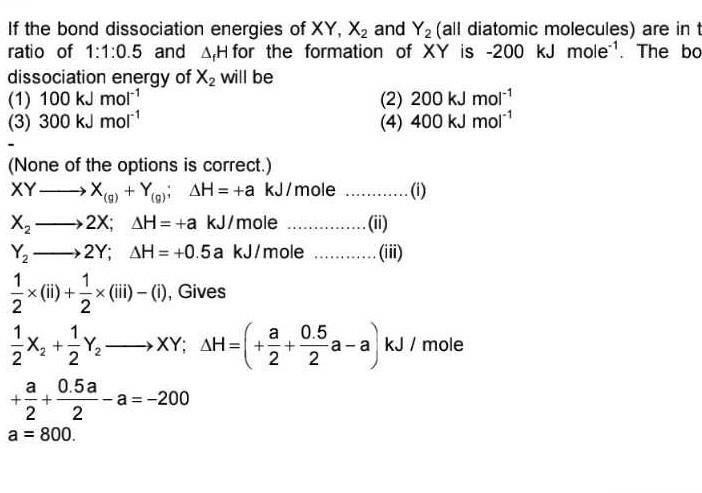QUESTION: 5

The standard enthalpy of formation (ΔfHº) at 298 K for methane, CH4(g), is -74.8 kJ mol-1. The additional information required to determine the average energy for C - H bond formation would be -

[AIEEE 2006]

Solution:

The correct answer is option D
Carbon is found in the solid-state. The state of the substance affects the enthalpy change.
Hence, the correct option is A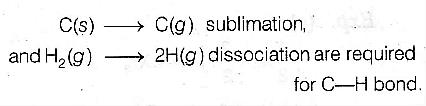QUESTION: 6

Oxidising power of chlorine in aqueous solution can be determined by the parameters indicated below :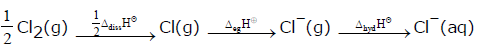The energy involved in the conversion of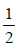Cl2(g) to Cl-(aq)

(using the data, Δdiss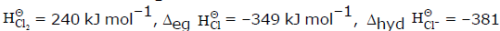kJ mol-1) will be -                      [AIEEE 2008]

Solution:

Oxidising power of chlorine in aqueous solution can be estimated by calculating the overall enthalpy change of reaction.
The reaction takes place with following steps:
1/2Cl2 —> Cl               ΔH1
Cl —> Cl−                    ΔH2
Cl− —> Cl− (aq)          ΔH3
ΔH will be the sum of the energy involved in these steps.
Hence, ΔH = ΔH1 +ΔH2 +ΔH3
ΔH1 = 240/2 = 120kJ/mol, ΔH2 = -349kJ/mol, ΔH3 = -381kJ/mol
Hence, ΔH = 120-349-381 = -610kJ/mol

QUESTION: 7

On the basis of the following thermochemical data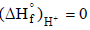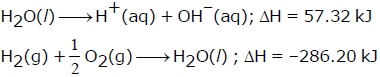The value of enthalpy of formation of OH- ion at 25ºC is -

[AIEEE 2009]

Solution:

The correct answer is option A
Given:-
H2​O(l)​⟶H+(aq.)​+OH(aq.)​ΔH=57.32kJ.....(1)
H2​(g)​+
1/2O2​(g)​⟶H2​O(l) ​ΔH=−286.20kJ.....(2)
H2​O(l)​+H2​(g)​+
1/2​O2​(g)​⟶H+(aq.)​+OH(aq.)​+H2​O(l)​;
ΔH=(57.32)+(−286.20)kJ
H2​(g)​+
1/2​O2​(g)​⟶H+(aq.)​+OH(aq.)​;ΔH=−228.88kJ
Hence the value of enthalpy of formation of OH ion at 25℃ is −228.88kJ.

QUESTION: 8

In a fuel cell methanol is used as fuel and oxygen gas is used as an oxidizer. The reaction is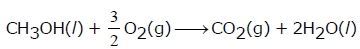At 298 K standard Gibb's energies of formation for CH3OH(l), H2O(l) and CO2(g) are -166.2,  -237.2 and -394.4 kJ mol-1 respectively. If standard enthalpy of combustion of methanol is -726 kJ mol-1, efficiency of the fuel cell will be-

[AIEEE 2009]

Solution:

CH3OH (l) + 3/2 O2 (g) → CO2 (g) + 2H2O(l)
∆Gr = ∆Gf(CO2,g) + 2∆Gf(H2O,l) - ∆Gf(CH3OH,l) - 3/2∆Gf(O2,g)
= -394.4 + 2(-237.2) - (-166.2) - 0
= -702.6 kJ
%age efficiency = 702.6/726 × 100 = 97%

QUESTION: 9

The value of enthalpy change (DH) for the reaction    C2H5OH(l) + 3O2(g) → 2CO2(g) + 3H2O(l)

at 27°C is -1366.5 kJ mol-1. The value of internal energy change for the above reaction at this temperature will be -

[AIEEE 2011]

Solution:

The correct answer is option B
The change in the number of moles of gaseous species  Δn = 2−3 =−1
ΔH = ΔE+ΔnRT
−1366.5 kJ/mol = ΔE+[(−1)×8.314×10−3 kJ/mol/K×(27+273) K]
ΔE = −1364.0 kJ/mol
The value of internal energy change for the reaction is −1364.0 kJ/mol.

QUESTION: 10

Consider the reaction :

4NO2(g) + O2(g) → 2N2O5(g), ΔrH = -111kJ If N2O5(s) is formed instead of N2O5(g) in the above reaction, the ΔrH value will be : (given, ΔH of sublimation for N2O5 is 54 kJ mol-1)

[AIEEE 2011]

Solution:

The correct answer is Option D.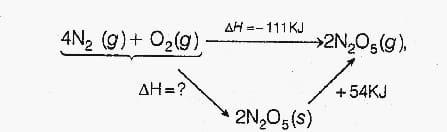From Hess’s Law;
ΔHf + ΔHsub =  ΔHreaction
ΔHf =  ΔHreaction - ΔHsub
=    - 111 kJ  -  54 kJ
=    - 165 kJ
Thus the enthalpy of the formation (ΔHf) for N2O5(s) is -165 kJ

QUESTION: 11

In view of the signs of DrG° for the following reactions :

PbO2 + Pb → 2 PbO, DrG° < 0

SnO2 + Sn → 2 SnO, DrG° > 0,

which oxidation states are more characteristic for lead and tin ?

[AIEEE 2011]

Solution: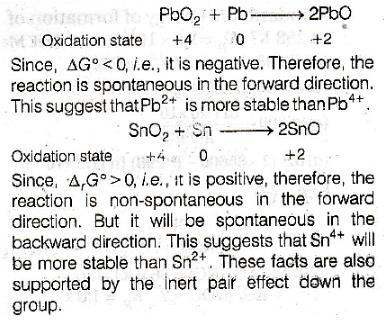QUESTION: 12

The standard enthalpy of formation of NH3 is -46.0 kJ mol-1 . If the enthalpy of formation of H2 from its atoms is -436 kJ mol-1 and that of N2 is -712 kJ mol-1 , the average bond enthalpy of N - H bond in

[AIEEE 2010]

Solution: